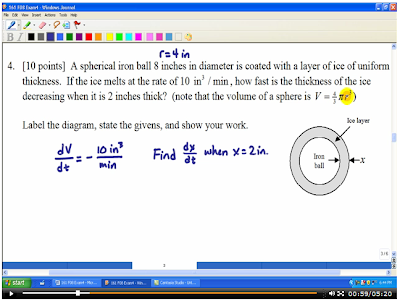So you’ve given a test in your calculus class – the dreaded test that covers related rates, optimization, and graphical analysis. You could spend the next class period going through the questions on the exam and answering questions – but if you do that, then that’s all you’ll do.

Let’s see … how can we go over the test without losing class time?Well, here’s how I’m doing it now – I record the making of the answer key.

1. Print the test to Windows Journal.
2. Record yourself explaining each problem (use Jing) while you write the answer key in Journal (or OneNote).
3. Produce each problem as a separate file, and load the files (or links to the files) into your online course shell.

Here’s one of the related rates problems.

And for the graphical analysis problem, I recorded a couple different versions of how you might have taken the derivatives for the problem (I needed them for grading anyways). In this case, I produced a separate video for each part (first derivative, 2nd derivative by log differentiation, 2nd derivative by quotient rule, etc.)